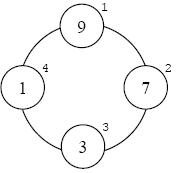favorite Нам необходимо немного Вашей помощи чтобы сайт продолжал работать, нажмите на банер чтобы узнать больше.
Задачи

# Проблема простых чиселOne of the most difficulties of an instructor is question design for the final-term exam. Dr. Ghavamnia teaches “Fundamentals of Algorithms” at University of Isfahan (UI) and has designed a good practical algorithmic problem for his exam. He wants that students use all of their algorithmic skills to code this problem as best and efficient as they can. The problem is as follows: a ring is composed of N circles numbered from 1 to N as shown in diagram. You are given N integer numbers. Put these numbers into each circle separately with the condition that sum of numbers in three adjacent circles must be a prime.

Each test case may have several solutions and your task is to find the one with minimum weighted sum. Weighted sum is defined as the sum of products of each number in circle by the sequence number of that circle. For instance, the weighted sum in above diagram is 36 (and also it is the minimum).

#### Input

The first line of input contains a single integer, the number of test cases. Following, there is one line for each test case. Each test case begins with one integer, N (3 ≤ N ≤ 15), which is the number of circles. Next N integers are the given numbers to put in circles (all between 1 and 100, inclusively).

#### Output

There should be one line for each test case in output. If there’s no way for building the ring, the word impossible should be outputted, or otherwise, a single integer which is the minimum weighted sum.

Лимит времени 4 секунды
Лимит использования памяти 64 MiB
Входные данные #1
1
15 11 13 15 17 19 21 22 23 25 27 29 31 33 35 37

Выходные данные #1
impossible